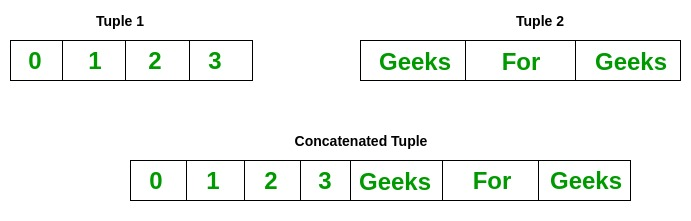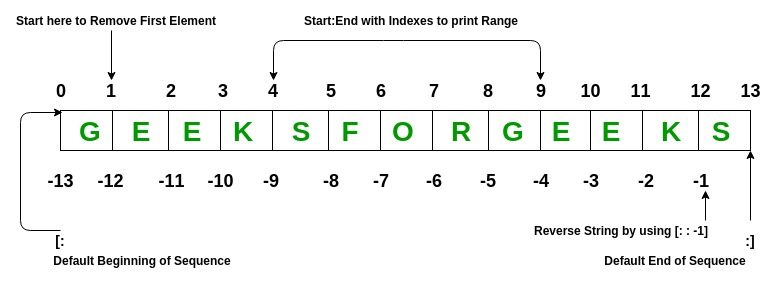# Python Tuples

Tuple is a collection of Python objects much like a list. The sequence of values stored in a tuple can be of any type, and they are indexed by integers.
Values of a tuple are syntactically separated by ‘commas’. Although it is not necessary, it is more common to define a tuple by closing the sequence of values in parentheses. This helps in understanding the Python tuples more easily.

## Creating a Tuple

In Python, tuples are created by placing sequence of values separated by ‘comma’ with or without the use of parentheses for grouping of data sequence.

Note – Creation of Python tuple without the use of parentheses is known as Tuple Packing.

#### Python program to demonstrate addition of elements in a Tuple.

 `#Creating an empty Tuple ` `Tuple1 ``=` `() ` `print``(``"Initial empty Tuple: "``) ` `print` `(Tuple1) ` ` `  `#Creatting a Tuple  ` `#with the use of string ` `Tuple1 ``=` `(``'Geeks'``, ``'For'``) ` `print``(``"\nTuple with the use of String: "``) ` `print``(Tuple1) ` ` `  `# Creating a Tuple with ` `# the use of list ` `list1 ``=` `[``1``, ``2``, ``4``, ``5``, ``6``] ` `print``(``"\nTuple using List: "``) ` `print``(``tuple``(list1)) ` ` `  `#Creating a Tuple  ` `#with the use of built-in function ` `Tuple1 ``=` `tuple``(``'Geeks'``) ` `print``(``"\nTuple with the use of function: "``) ` `print``(Tuple1) `

Output:

```Initial empty Tuple:
()

Tuple with the use of String:
('Geeks', 'For')

Tuple using List:
(1, 2, 4, 5, 6)

Tuple with the use of function:
('G', 'e', 'e', 'k', 's')
```

#### Creating a Tuple with Mixed Datatypes.

Tuples can contain any number of elements and of any datatype (like strings, integers, list, etc.). Tuples can also be created with a single element, but it is a bit tricky. Having one element in the parentheses is not sufficient, there must be a trailing ‘comma’ to make it a tuple.

 `#Creating a Tuple ` `#with Mixed Datatype ` `Tuple1 ``=` `(``5``, ``'Welcome'``, ``7``, ``'Geeks'``) ` `print``(``"\nTuple with Mixed Datatypes: "``) ` `print``(Tuple1) ` ` `  `#Creating a Tuple ` `#with nested tuples ` `Tuple1 ``=` `(``0``, ``1``, ``2``, ``3``) ` `Tuple2 ``=` `(``'python'``, ``'geek'``) ` `Tuple3 ``=` `(Tuple1, Tuple2) ` `print``(``"\nTuple with nested tuples: "``) ` `print``(Tuple3) ` ` `  `#Creating a Tuple ` `#with repetition ` `Tuple1 ``=` `(``'Geeks'``,) ``*` `3` `print``(``"\nTuple with repetition: "``) ` `print``(Tuple1) ` ` `  `#Creating a Tuple  ` `#with the use of loop ` `Tuple1 ``=` `(``'Geeks'``) ` `n ``=` `5` `print``(``"\nTuple with a loop"``) ` `for` `i ``in` `range``(``int``(n)): ` `    ``Tuple1 ``=` `(Tuple1,) ` `    ``print``(Tuple1) `

Output:

```Tuple with Mixed Datatypes:
(5, 'Welcome', 7, 'Geeks')

Tuple with nested tuples:
((0, 1, 2, 3), ('python', 'geek'))

Tuple with repetition:
('Geeks', 'Geeks', 'Geeks')

Tuple with a loop
('Geeks',)
(('Geeks',),)
((('Geeks',),),)
(((('Geeks',),),),)
((((('Geeks',),),),),)
```

## Accessing of Tuples

Tuples are immutable, and usually, they contain a sequence of heterogeneous elements that are accessed via unpacking or indexing (or even by attribute in the case of named tuples). Lists are mutable, and their elements are usually homogeneous and are accessed by iterating over the list.

NOTE : In unpacking of tuple number of variables on left hand side should be equal to number of values in given tuple a.

 `#Accessing Tuple ` `#with Indexing ` `Tuple1 ``=` `tuple``(``"Geeks"``) ` `print``(``"\nFirst element of Tuple: "``) ` `print``(Tuple1[``1``]) ` ` `  ` `  `#Tuple unpacking ` `Tuple1 ``=` `(``"Geeks"``, ``"For"``, ``"Geeks"``) ` ` `  `#This line unpack  ` `#values of Tuple1 ` `a, b, c ``=` `Tuple1 ` `print``(``"\nValues after unpacking: "``) ` `print``(a) ` `print``(b) ` `print``(c) `

Output:

```First element of Tuple:
e

Values after unpacking:
Geeks
For
Geeks```

## Concatenation of Tuples

Concatenation of tuple is the process of joining of two or more Tuples. Concatenation is done by the use of ‘+’ operator. Concatenation of tuples is done always from the end of the original tuple. Other arithmetic operations do not apply on Tuples.
Note- Only same datatypes can be combined with concatenation, an error arises if a list and a tuple are combined.`# Concatenaton of tuples ` `Tuple1 ``=` `(``0``, ``1``, ``2``, ``3``) ` `Tuple2 ``=` `(``'Geeks'``, ``'For'``, ``'Geeks'``) ` ` `  `Tuple3 ``=` `Tuple1 ``+` `Tuple2 ` ` `  `# Printing first Tuple ` `print``(``"Tuple 1: "``) ` `print``(Tuple1) ` ` `  `# Printing Second Tuple ` `print``(``"\nTuple2: "``) ` `print``(Tuple2) ` ` `  `# Printing Final Tuple ` `print``(``"\nTuples after Concatenaton: "``) ` `print``(Tuple3) `

Output:

```Tuple 1:
(0, 1, 2, 3)

Tuple2:
('Geeks', 'For', 'Geeks')

Tuples after Concatenaton:
(0, 1, 2, 3, 'Geeks', 'For', 'Geeks')
```

## Slicing of Tuple

Slicing of a Tuple is done to fetch a specific range or slice of sub-elements from a Tuple. Slicing can also be done to lists and arrays. Indexing in a list results to fetching a single element whereas Slicing allows to fetch a set of elements.
Note- Negative Increment values can also be used to reverse the sequence of Tuples`# Slicing of a Tuple ` ` `  `# Slicing of a Tuple ` `# with Numbers ` `Tuple1 ``=` `tuple``(``'GEEKSFORGEEKS'``) ` ` `  `# Removing First element ` `print``(``"Removal of First Element: "``) ` `print``(Tuple1[``1``:]) ` ` `  `# Reversing the Tuple  ` `print``(``"\nTuple after sequence of Element is reversed: "``) ` `print``(Tuple1[::``-``1``]) ` ` `  `# Printing elements of a Range ` `print``(``"\nPrinting elements between Range 4-9: "``) ` `print``(Tuple1[``4``:``9``]) `

Output:

```Removal of First Element:
('E', 'E', 'K', 'S', 'F', 'O', 'R', 'G', 'E', 'E', 'K', 'S')

Tuple after sequence of Element is reversed:
('S', 'K', 'E', 'E', 'G', 'R', 'O', 'F', 'S', 'K', 'E', 'E', 'G')

Printing elements between Range 4-9:
('S', 'F', 'O', 'R', 'G')
```

## Deleting a Tuple

Tuples are immutable and hence they do not allow deletion of a part of it. Entire tuple gets deleted by the use of del() method.
Note- Printing of Tuple after deletion results to an Error.

 `# Deleting a Tuple ` ` `  `Tuple1 ``=` `(``0``, ``1``, ``2``, ``3``, ``4``) ` `del` `Tuple1 ` ` `  `print``(Tuple1) `

Traceback (most recent call last):
File “/home/efa50fd0709dec08434191f32275928a.py”, line 7, in
print(Tuple1)
NameError: name ‘Tuple1’ is not defined

#### Built-In Methods

Built-in Function Description
all() Returns true if all element are true or if tuple is empty
any() return true if any element of the tuple is true. if tuple is empty, return false
len() Returns length of the tuple or size of the tuple
enumerate() Returns enumerate object of tuple
max() return maximum element of given tuple
min() return minimum element of given tuple
sum() Sums up the numbers in the tuple
sorted() input elements in the tuple and return a new sorted list
tuple() Convert an iterable to a tuple.

### Recent Articles on Tuple

Tuples Programs

My Personal Notes arrow_drop_up

Improved By : nikhilaggarwal3, awpkalsi

Article Tags :

20

Please write to us at contribute@geeksforgeeks.org to report any issue with the above content.